Next: 8.5 Change of Scale Up: 8. Integrable Functions Previous: 8.3 A Non-integrable Function   Index

# 8.4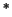The Ruler Function

8.38   Example (Ruler function.) We now present an example of an integrable function that is not monotonic on any interval of positive length. Define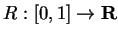by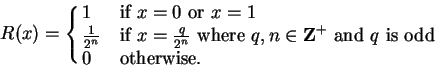This formula defines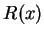uniquely: If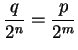where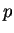and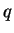are odd, then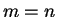. (If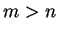, we get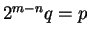, which says that an even number is odd.) The set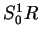under the graph of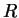is shown in the figure.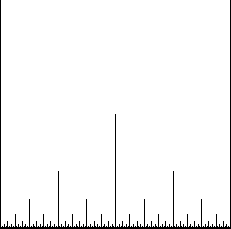This set resembles the markings giving fractions of an inch on a ruler, which motivates the name ruler function for. It is easy to see thatis not monotonic on any interval of length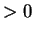. For each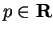let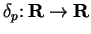be defined by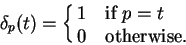We have seen that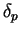is integrable on any interval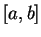and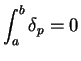. Now define a sequence of functions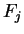by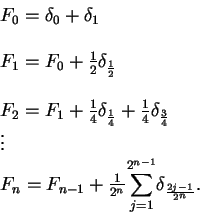Each functionis integrable with integral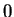and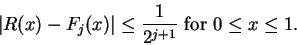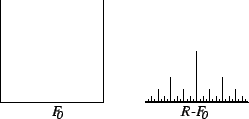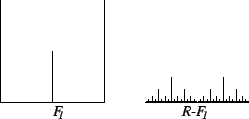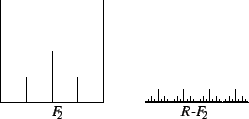I will now show thatis integrable.

Let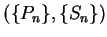be a partition-sample sequence for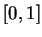. I'll show that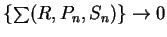.

Let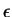be a generic element in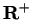. Observe that if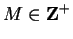then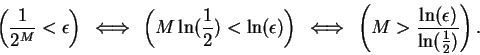Hence by the Archimedian property, we can chooseso that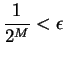. Then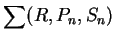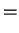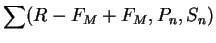(8.39)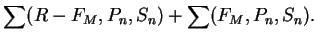(8.40)

Now since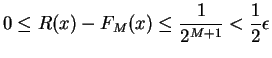for all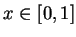, we have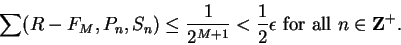Since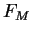is integrable and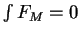, we have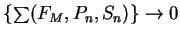so there is an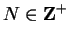such that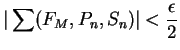for all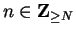. By equation (8.40) we have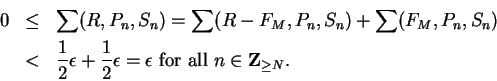Hence, and henceis integrable and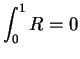.

8.41   Exercise. A Letbe the ruler function. We just gave a complicated proof thatis integrable and. Explain why if you assumeis integrable, then it is easy to show that.

Also show that if you assume that the non-integrable function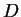in equation (8.37) is integrable then it is easy to show that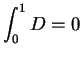.Next: 8.5 Change of Scale Up: 8. Integrable Functions Previous: 8.3 A Non-integrable Function   Index
Ray Mayer 2007-09-07